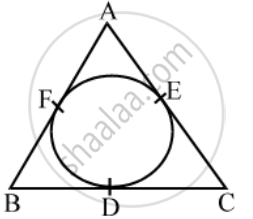# In the given figure, the incircle of ∆ABC touches the sides BC, CA and AB at D, E, F respectively. Prove that AF + BD + CE = AE + CD + BF = 1/2(perimeter of ΔABC) - Mathematics

Sum

In the given figure, the incircle of ∆ABC touches the sides BC, CA and AB at D, E, F respectively. Prove that AF + BD + CE = AE + CD + BF = \frac { 1 }{ 2 } ("perimeter of ∆ABC")

#### Solution

We know that the lengths of tangents from an exterior point to a circle are equal.∴ AF = AE …. (i) [tangents from A]

BD = BF ….. (ii) [tangents from B]

CE =CD …. (iii) [tangents from C]

Adding (i), (ii) and (iii), we get

(AF + BD + CE) = (AE + BF + CD) = k (say)

Perimeter of ∆ABC = (AF + BD +CE) + (AE + BF + CD)

= (k + k) = 2k

∴ k = \frac { 1 }{ 2 } "(perimeter of ∆ABC)"

Hence AF + BD + CE = AE + CD + BF = \frac { 1 }{ 2 } ("perimeter of ∆ABC")

Concept: Concept of Circle - Centre, Radius, Diameter, Arc, Sector, Chord, Segment, Semicircle, Circumference, Interior and Exterior, Concentric Circles
Is there an error in this question or solution?
Share